Add & Subtract Within 20 Math Video for Kids - K, 1st, & 2nd Grades
1%
It was processed successfully!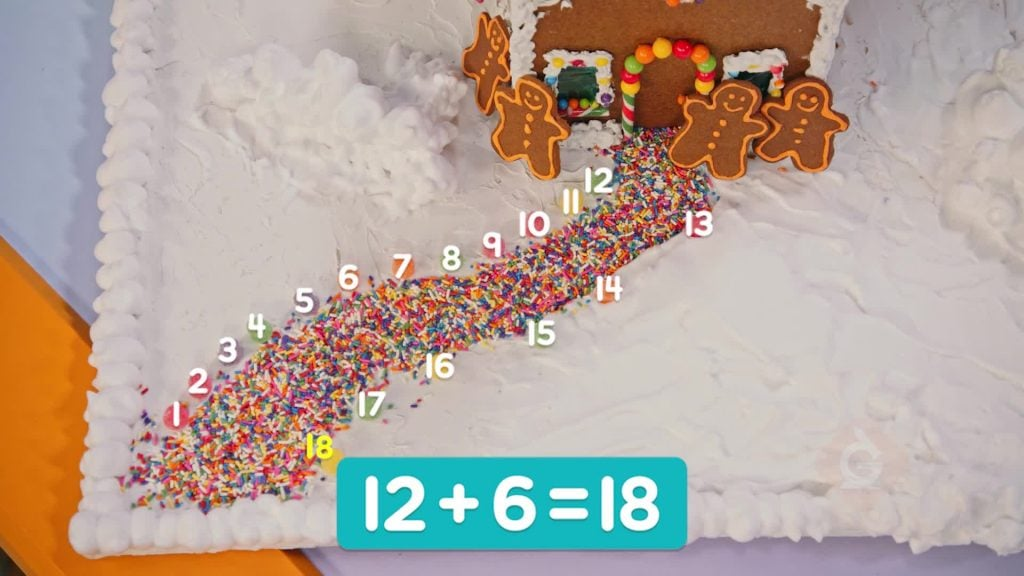HOW CAN YOU ADD & SUBTRACT?

When you add 2 numbers, you can count all to find the total. You can also count on from one number. To subtract, you can count on or count back.

To better understand adding & subtracting within 20…

HOW CAN YOU ADD & SUBTRACT?. When you add 2 numbers, you can count all to find the total. You can also count on from one number. To subtract, you can count on or count back. To better understand adding & subtracting within 20…

## LET’S BREAK IT DOWN!

### Count all to find the number of hats.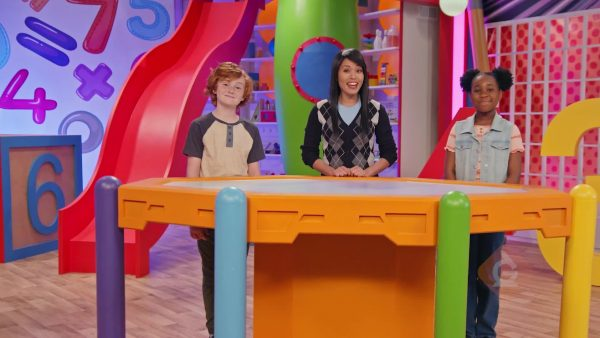There are 4 red hats. There are 9 blue hats. Count all to find how many hats in all. Count the red hats: 1, 2, 3, 4. Count the blue hats: 5, 6, 7, 8, 9, 10, 11, 12, 13. There are 13 hats in all.

Count all to find the number of hats. There are 4 red hats. There are 9 blue hats. Count all to find how many hats in all. Count the red hats: 1, 2, 3, 4. Count the blue hats: 5, 6, 7, 8, 9, 10, 11, 12, 13. There are 13 hats in all.

### Count on to find the number of gumdrops.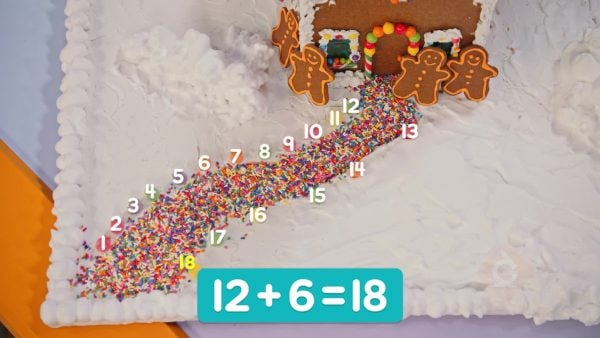You put 12 gumdrops on a gingerbread house. Gavin put on 6 gumdrops. You can count all to find that there are 18 gumdrops in all. Or you can start at 12 gumdrops and count on 6 more: 13, 14, 15, 16, 17, 18 gumdrops. 12 + 6 = 18.

Count on to find the number of gumdrops. You put 12 gumdrops on a gingerbread house. Gavin put on 6 gumdrops. You can count all to find that there are 18 gumdrops in all. Or you can start at 12 gumdrops and count on 6 more: 13, 14, 15, 16, 17, 18 gumdrops. 12 + 6 = 18.

### Count on to find a missing number.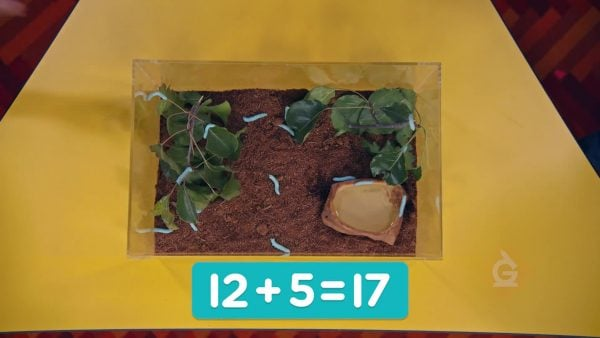There were 12 caterpillars on a tree. Now there are 17 caterpillars. How many more caterpillars joined? 12 plus how many is 17? 12 + ? = 17. Start at 12 and count on to 17. Put your fingers up one at a time as you count: 13, 14, 15, 16, 17. You put 5 fingers up. 5 caterpillars joined. 12 + 5 = 17.

Count on to find a missing number. There were 12 caterpillars on a tree. Now there are 17 caterpillars. How many more caterpillars joined? 12 plus how many is 17? 12 + ? = 17. Start at 12 and count on to 17. Put your fingers up one at a time as you count: 13, 14, 15, 16, 17. You put 5 fingers up. 5 caterpillars joined. 12 + 5 = 17.

### Count back to find how many more trophies.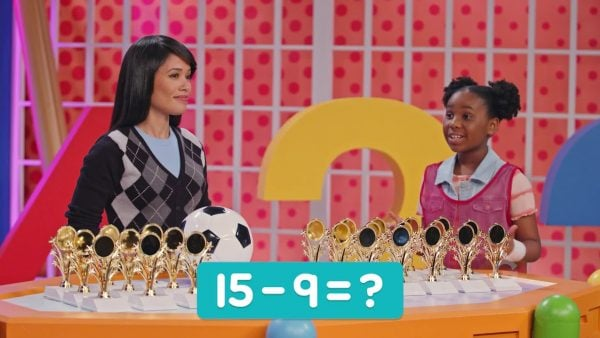There are 15 trophies. There are 9 medals. How many more trophies are there than medals? Subtract 15 – 9. You can start with 15 and count back to 9: 14, 13, 12, 11, 10, 9. You counted back 6. There are 6 more trophies than medals.

Count back to find how many more trophies. There are 15 trophies. There are 9 medals. How many more trophies are there than medals? Subtract 15 – 9. You can start with 15 and count back to 9: 14, 13, 12, 11, 10, 9. You counted back 6. There are 6 more trophies than medals.

### Count back to find a missing number.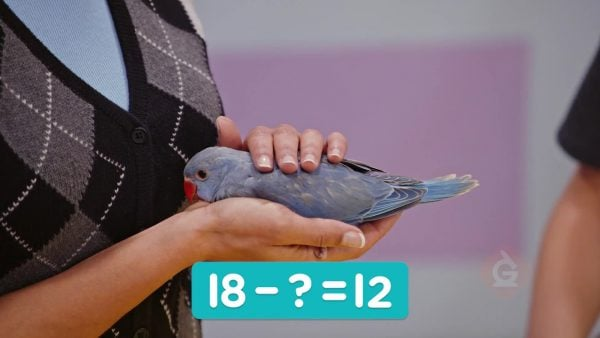A parrot had 18 seeds. She ate some of the seeds. Now the parrot has 12 seeds left. How many seeds did the parrot eat? Start at 18 and count back to 12: 17, 16, 15, 14, 13, 12. You counted back 6 numbers. The parrot ate 6 seeds.

Count back to find a missing number. A parrot had 18 seeds. She ate some of the seeds. Now the parrot has 12 seeds left. How many seeds did the parrot eat? Start at 18 and count back to 12: 17, 16, 15, 14, 13, 12. You counted back 6 numbers. The parrot ate 6 seeds.

## ADD & SUBTRACT WITHIN 20 VOCABULARY

To put together.
Subtract
To take away.
Count all
To count every object. One way to add is to count all.
Count on
To count in increasing order. You can use counting on to solve addition and subtraction problems. For example, you can add 6 + 3 by starting from 6 and then counting on 3: 7, 8, 9.
Count back
To count in decreasing order. You can use counting back to solve addition and subtraction problems. For example, you can subtract 6 – 3 by starting from 6 and then counting back 3: 5, 4, 3.
Difference
The result of subtracting.

## ADD & SUBTRACT WITHIN 20 DISCUSSION QUESTIONS

### How can you add 5 + 9?

You could count all, count on from 5, or count on from 9. 5 + 9 = 14.

### In an addition problem like 9 + ? = 12, how can you find the missing number?

I can count on to find the missing number. Start at 9 and count on to 12. I put up one finger for each number I count. 10, 11, 12. I put up 3 fingers, which means I counted on 3. So, the missing number is 3.

### How can you subtract 15 – 3?

I could count back from 15 or count on from 3. 15 – 3 = 12.

### In a subtraction problem like 19 – ? = 14, how can you find the missing number?

I can count back to find the missing number. Start at 19 and count back to 14. To keep track on my fingers, I put up one finger for each number I count. 18, 17, 16, 15, 14. I put up 5 fingers, so I counted back 5. So, the missing number is 5.

### You have 14 stickers. You give some away. You have 9 left. How many stickers did you give away?

14 – ? = 9. Start from 14. Then, count back to 9. 13, 12, 11, 10, 9. I counted back 5 numbers, so the missing number is 5. I gave away 5 stickers.
X

## Success

We’ve sent you an email with instructions how to reset your password.
Ok